# Exercises A pdfLoading previews...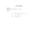calculate div and curl for a vector field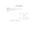calculate div and curl for a vector field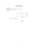calculate div and curl for a vector field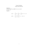calculate div and curl for a vector field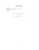calculate div and curl for a vector field
13 files in this resource

## Exercises A pdf

Exercises and solutions in PDFView Item